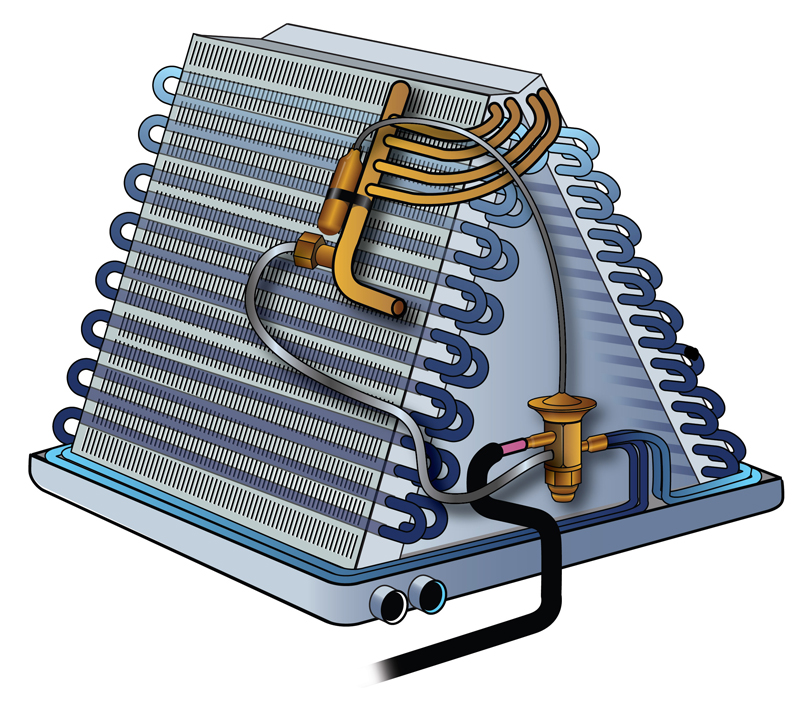## Basic Electrical Theory

1. According to Ohm’s Law, increasing resistance in a circuit

Question 1 of 10

2. A 5KW heatstrip rated at 230V would draw how many amps at 23V?

Question 2 of 10

3. Electrons contain a _________ Charge

Question 3 of 10

4. Atoms with _______ electrons in the outer layer (Valance) generally make better Insulators

Question 4 of 10

5. Volts X Amps = ________

Question 5 of 10

6. A common example of an inductive load is

Question 6 of 10

7. 1 Volt is

Question 7 of 10

8. A _________ is an example of a switch

Question 8 of 10

9. 1 HP = _______ BTUs per Hr

Question 9 of 10

10. When you install a dimmer on a incandescent light bulb and add resistance to the circuit the light will dim and the amperage of the circuit will decrease.

Question 10 of 10

1.Jonathan Hoeppner says:

I though P=EI and E=IR. To #5.

1.Bryan Orr says:

Watts(P) = Amps(I) X Volts (E)

2.Martin M says:

Question number 2: I = P / U gives 5000W / 23V = 217 A

No?

3.Jamie Kitchen says:

746 watts per hp. Should have read the question more closely and realized it was asking for Btu’s.

4.Taylor Kristiansen says:

For Question #2 can you please explain how you got to that answer!? 1 Kw = 1000W, so using P= E x I, I just did 5000W (5Kw) / 23 = 217 .. I’m super confused!

1.Michael Liddle says:

Instead of doing P=V x A. We convert 5 kW to 5000 watts. Divide 5000W / 230V = nominally 22 amps (21.73). We can now use ohms law to find the actual resistance of the 5kW strip with this information.

R= V/A: R= 230V/21.73A. R=10.58
now if we do 23V/10.58 we get 2.17 amps.

So the answer is really found in finding the resistance in the 5kW strip.

This site uses Akismet to reduce spam. Learn how your comment data is processed.

Scroll to top
Translate »

### Daily Tech Tip

Get the (near) daily Tech Tip email right in your inbox!